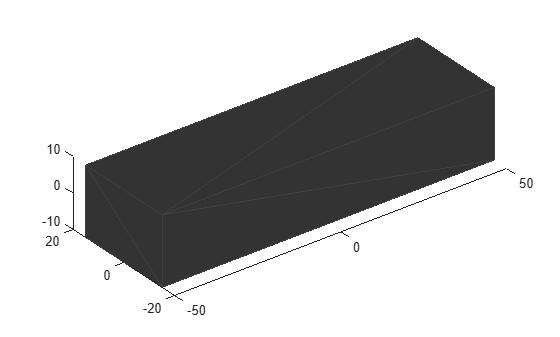# scale

Scale mesh in each dimension

## Syntax

``scaledMesh = scale(mesh,scaleFactor)``
``scaledMesh = scale(mesh,[sx sy sz])``

## Description

example

````scaledMesh = scale(mesh,scaleFactor)` scales the object mesh by `scaleFactor`. `scaleFactor` can be the same for all dimensions or defined separately as elements of a 1-by-3 vector in the order x, y, and z.```
````scaledMesh = scale(mesh,[sx sy sz])` scales the object mesh along the dimensions x, y, and z by the scaling factors `sx`, `sy`, and `sz`.```

## Examples

collapse all

Create an `extendedObjectMesh` object and scale the object.

Construct a cuboid mesh of unit dimensions.

` cuboid = extendedObjectMesh('cuboid');`

Scale the mesh by different factors along each of the three axes.

`scaledCuboid = scale(cuboid,[100 30 20]);`

Visualize the mesh.

`show(scaledCuboid);`## Input Arguments

collapse all

Extended object mesh, specified as an `extendedObjectMesh` object.

Scaling factor for the object mesh, specified as a positive real scalar or as a 1-by-3 vector in the order x, y, and z.

Data Types: `single` | `double`

Scaling factor for x-axis, specified as a positive real scalar.

Data Types: `single` | `double`

Scaling factor for y-axis, specified as a positive real scalar.

Data Types: `single` | `double`

Scaling factor for z-axis, specified as a positive real scalar.

Data Types: `single` | `double`

## Output Arguments

collapse all

Scaled object mesh, returned as an `extendedObjectMesh` object.

## Version History

Introduced in R2020b